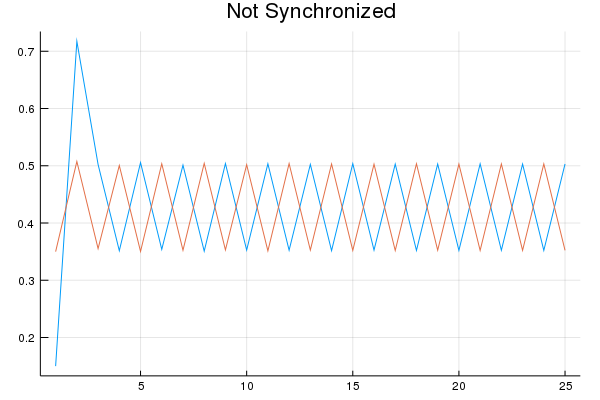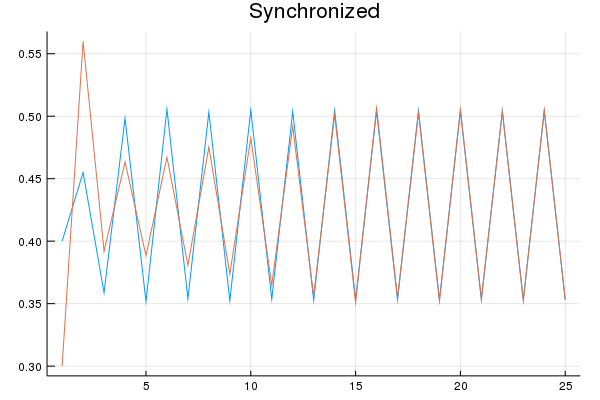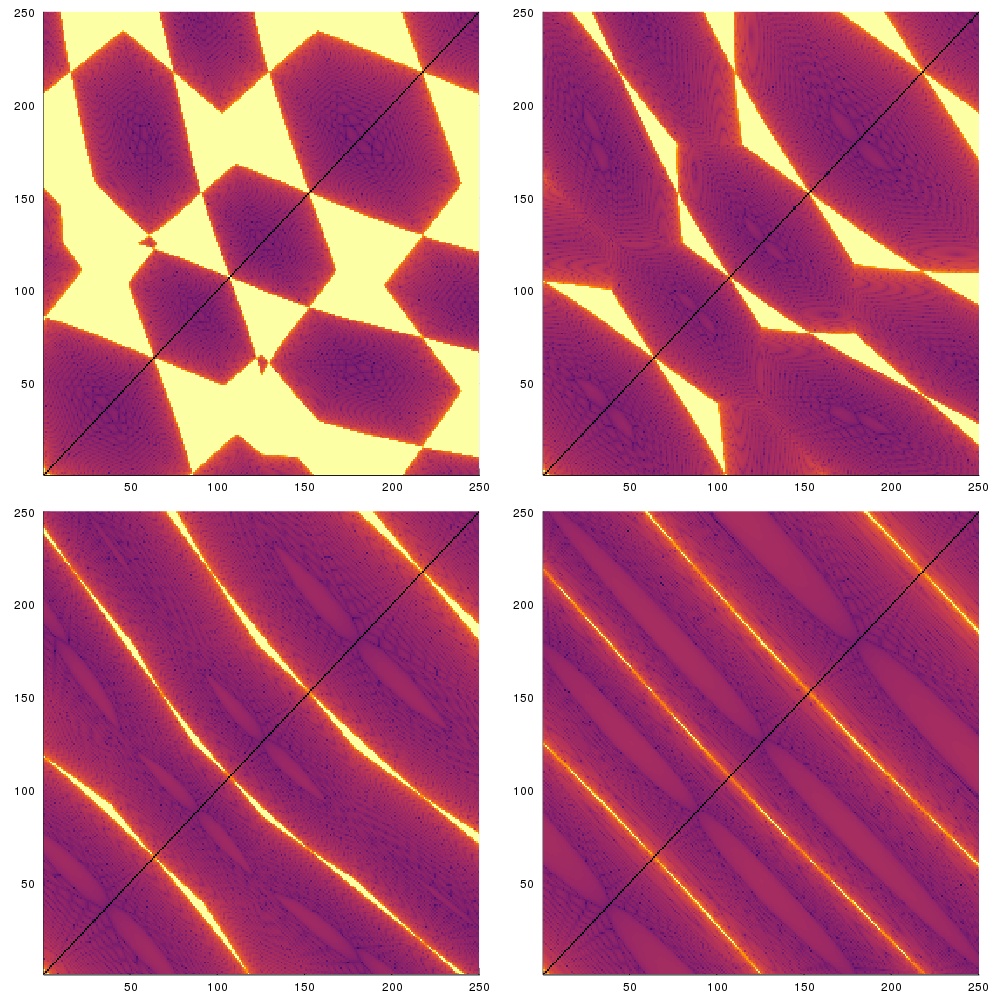We are working to support a site-wide PDF but it is not yet available. You can download PDFs for individual lectures through the download badge on each lecture page.How to read this lecture...

Code should execute sequentially if run in a Jupyter notebook

• See the set up page to install Jupyter, Julia (1.0+) and all necessary libraries
• Please direct feedback to contact@quantecon.org or the discourse forum
• For some notebooks, enable content with "Trust" on the command tab of Jupyter lab
• If using QuantEcon lectures for the first time on a computer, execute ] add InstantiateFromURL inside of a notebook or the REPL

# Globalization and Cycles¶

## Contents¶

Co-authored with Chase Coleman

## Overview¶

In this lecture, we review the paper Globalization and Synchronization of Innovation Cycles by Kiminori Matsuyama, Laura Gardini and Iryna Sushko

This model helps us understand several interesting stylized facts about the world economy

One of these is synchronized business cycles across different countries

Most existing models that generate synchronized business cycles do so by assumption, since they tie output in each country to a common shock

They also fail to explain certain features of the data, such as the fact that the degree of synchronization tends to increase with trade ties

By contrast, in the model we consider in this lecture, synchronization is both endogenous and increasing with the extent of trade integration

In particular, as trade costs fall and international competition increases, innovation incentives become aligned and countries synchronize their innovation cycles

### Background¶

The model builds on work by Judd [Jud85], Deneckner and Judd [DJ92] and Helpman and Krugman [HK85] by developing a two country model with trade and innovation

On the technical side, the paper introduces the concept of coupled oscillators to economic modeling

As we will see, coupled oscillators arise endogenously within the model

Below we review the model and replicate some of the results on synchronization of innovation across countries

## Key Ideas¶

It is helpful to begin with an overview of the mechanism

### Innovation Cycles¶

As discussed above, two countries produce and trade with each other

In each country, firms innovate, producing new varieties of goods and, in doing so, receiving temporary monopoly power

Imitators follow and, after one period of monopoly, what had previously been new varieties now enter competitive production

Firms have incentives to innovate and produce new goods when the mass of varieties of goods currently in production is relatively low

In addition, there are strategic complementarities in the timing of innovation

Firms have incentives to innovate in the same period, so as to avoid competing with substitutes that are competitively produced

This leads to temporal clustering in innovations in each country

After a burst of innovation, the mass of goods currently in production increases

However, goods also become obsolete, so that not all survive from period to period

This mechanism generates a cycle, where the mass of varieties increases through simultaneous innovation and then falls through obsolescence

### Synchronization¶

In the absence of trade, the timing of innovation cycles in each country is decoupled

This will be the case when trade costs are prohibitively high

If trade costs fall, then goods produced in each country penetrate each other’s markets

As illustrated below, this leads to synchonization of business cycles across the two countries

## Model¶

Let’s write down the model more formally

(The treatment is relatively terse since full details can be found in the original paper)

Time is discrete with $t = 0, 1, \dots$

There are two countries indexed by $j$ or $k$

In each country, a representative household inelastically supplies $L_j$ units of labor at wage rate $w_{j, t}$

Without loss of generality, it is assumed that $L_{1} \geq L_{2}$

Households consume a single nontradeable final good which is produced competitively

Its production involves combining two types of tradeable intermediate inputs via

$$Y_{k, t} = C_{k, t} = \left( \frac{X^o_{k, t}}{1 - \alpha} \right)^{1-\alpha} \left( \frac{X_{k, t}}{\alpha} \right)^{\alpha}$$

Here $X^o_{k, t}$ is a homogeneous input which can be produced from labor using a linear, one-for-one technology

It is freely tradeable, competitively supplied, and homogeneous across countries

By choosing the price of this good as numeraire and assuming both countries find it optimal to always produce the homogeneous good, we can set $w_{1, t} = w_{2, t} = 1$

The good $X_{k, t}$ is a composite, built from many differentiated goods via

$$X_{k, t}^{1 - \frac{1}{\sigma}} = \int_{\Omega_t} \left[ x_{k, t}(\nu) \right]^{1 - \frac{1}{\sigma}} d \nu$$

Here $x_{k, t}(\nu)$ is the total amount of a differentiated good $\nu \in \Omega_t$ that is produced

The parameter $\sigma > 1$ is the direct partial elasticity of substitution between a pair of varieties and $\Omega_t$ is the set of varieties available in period $t$

We can split the varieties into those which are supplied competitively and those supplied monopolistically; that is, $\Omega_t = \Omega_t^c + \Omega_t^m$

### Prices¶

Demand for differentiated inputs is

$$x_{k, t}(\nu) = \left( \frac{p_{k, t}(\nu)}{P_{k, t}} \right)^{-\sigma} \frac{\alpha L_k}{P_{k, t}}$$

Here

• $p_{k, t}(\nu)$ is the price of the variety $\nu$ and
• $P_{k, t}$ is the price index for differentiated inputs in $k$, defined by
$$\left[ P_{k, t} \right]^{1 - \sigma} = \int_{\Omega_t} [p_{k, t}(\nu) ]^{1-\sigma} d\nu$$

The price of a variety also depends on the origin, $j$, and destination, $k$, of the goods because shipping varieties between countries incurs an iceberg trade cost $\tau_{j,k}$

Thus the effective price in country $k$ of a variety $\nu$ produced in country $j$ becomes $p_{k, t}(\nu) = \tau_{j,k} \, p_{j, t}(\nu)$

Using these expressions, we can derive the total demand for each variety, which is

$$D_{j, t}(\nu) = \sum_k \tau_{j, k} x_{k, t}(\nu) = \alpha A_{j, t}(p_{j, t}(\nu))^{-\sigma}$$

where

$$A_{j, t} := \sum_k \frac{\rho_{j, k} L_{k}}{(P_{k, t})^{1 - \sigma}} \quad \text{and} \quad \rho_{j, k} = (\tau_{j, k})^{1 - \sigma} \leq 1$$

It is assumed that $\tau_{1,1} = \tau_{2,2} = 1$ and $\tau_{1,2} = \tau_{2,1} = \tau$ for some $\tau > 1$, so that

$$\rho_{1,2} = \rho_{2,1} = \rho := \tau^{1 - \sigma} < 1$$

The value $\rho \in [0, 1)$ is a proxy for the degree of globalization

Producing one unit of each differentiated variety requires $\psi$ units of labor, so the marginal cost is equal to $\psi$ for $\nu \in \Omega_{j, t}$

Additionally, all competitive varieties will have the same price (because of equal marginal cost), which means that, for all $\nu \in \Omega^c$,

$$p_{j, t}(\nu) = p_{j, t}^c := \psi \quad \text{and} \quad D_{j, t} = y_{j, t}^c := \alpha A_{j, t} (p_{j, t}^c)^{-\sigma}$$

Monopolists will have the same marked-up price, so, for all $\nu \in \Omega^m$ ,

$$p_{j, t}(\nu) = p_{j, t}^m := \frac{\psi }{1 - \frac{1}{\sigma}} \quad \text{and} \quad D_{j, t} = y_{j, t}^m := \alpha A_{j, t} (p_{j, t}^m)^{-\sigma}$$

Define

$$\theta := \frac{p_{j, t}^c}{p_{j, t}^m} \frac{y_{j, t}^c}{y_{j, t}^m} = \left(1 - \frac{1}{\sigma} \right)^{1-\sigma}$$

Using the preceding definitions and some algebra, the price indices can now be rewritten as

$$\left(\frac{P_{k,t}}{\psi}\right)^{1-\sigma} = M_{k,t} + \rho M_{j,t} \quad \text{where} \quad M_{j,t} := N_{j,t}^c + \frac{N_{j,t}^m}{ \theta}$$

The symbols $N_{j, t}^c$ and $N_{j, t}^m$ will denote the measures of $\Omega^c$ and $\Omega^m$ respectively

### New Varieties¶

To introduce a new variety, a firm must hire $f$ units of labor per variety in each country

Monopolist profits must be less than or equal to zero in expectation, so

$$N_{j,t}^m \geq 0, \quad \pi_{j, t}^m := (p_{j, t}^m - \psi) y_{j, t}^m - f \leq 0 \quad \text{and} \quad \pi_{j, t}^m N_{j,t}^m = 0$$

With further manipulations, this becomes

$$N_{j,t}^m = \theta(M_{j,t} - N_{j,t}^c) \geq 0, \quad \frac{1}{\sigma} \left[ \frac{\alpha L_j}{\theta(M_{j,t} + \rho M_{k,t})} + \frac{\alpha L_k}{\theta(M_{j,t} + M_{k,t} / \rho)} \right] \leq f$$

### Law of Motion¶

With $\delta$ as the exogenous probability of a variety becoming obsolete, the dynamic equation for the measure of firms becomes

$$N_{j, t+1}^c = \delta (N_{j, t}^c + N_{j, t}^m) = \delta (N_{j, t}^c + \theta(M_{j, t} - N_{j, t}^c))$$

We will work with a normalized measure of varieties

$$n_{j, t} := \frac{\theta \sigma f N_{j, t}^c}{\alpha (L_1 + L_2)}, \quad i_{j, t} := \frac{\theta \sigma f N_{j, t}^m}{\alpha (L_1 + L_2)}, \quad m_{j, t} := \frac{\theta \sigma f M_{j, t}}{\alpha (L_1 + L_2)} = n_{j, t} + \frac{i_{j, t}}{\theta}$$

We also use $s_j := \frac{L_j}{L_1 + L_2}$ to be the share of labor employed in country $j$

We can use these definitions and the preceding expressions to obtain a law of motion for $n_t := (n_{1, t}, n_{2, t})$

In particular, given an initial condition, $n_0 = (n_{1, 0}, n_{2, 0}) \in \mathbb{R}_{+}^{2}$, the equilibrium trajectory, $\{ n_t \}_{t=0}^{\infty} = \{ (n_{1, t}, n_{2, t}) \}_{t=0}^{\infty}$, is obtained by iterating on $n_{t+1} = F(n_t)$ where $F : \mathbb{R}_{+}^{2} \rightarrow \mathbb{R}_{+}^{2}$ is given by

\begin{aligned} F(n_t) &= \begin{cases} \big( \delta (\theta s_1(\rho) + (1-\theta) n_{1, t}), \delta (\theta s_2(\rho) + (1-\theta) n_{2, t}) \big) \; & \text{for } n_t \in D_{LL} \\ \big( \delta n_{1, t}, \delta n_{2, t} \big) \; &\text{for } n_t \in D_{HH} \\ \big( \delta n_{1, t}, \delta (\theta h_2(n_{1, t}) + (1-\theta) n_{2, t}) \big) &\text{for } n_t \in D_{HL} \\ \big( \delta (\theta h_1(n_{2, t}) + (1-\theta) n_{1, t}, \delta n_{2, t}) \big) &\text{for } n_t \in D_{LH} \end{cases} \end{aligned}

Here

\begin{aligned} D_{LL} & := \{ (n_1, n_2) \in \mathbb{R}_{+}^{2} | n_j \leq s_j(\rho) \} \\ D_{HH} & := \{ (n_1, n_2) \in \mathbb{R}_{+}^{2} | n_j \geq h_j(\rho) \} \\ D_{HL} & := \{ (n_1, n_2) \in \mathbb{R}_{+}^{2} | n_1 \geq s_1(\rho) \text{ and } n_2 \leq h_2(n_1) \} \\ D_{LH} & := \{ (n_1, n_2) \in \mathbb{R}_{+}^{2} | n_1 \leq h_1(n_2) \text{ and } n_2 \geq s_2(\rho) \} \end{aligned}

while

$$s_1(\rho) = 1 - s_2(\rho) = \min \left\{ \frac{s_1 - \rho s_2}{1 - \rho}, 1 \right\}$$

and $h_j(n_k)$ is defined implicitly by the equation

$$1 = \frac{s_j}{h_j(n_k) + \rho n_k} + \frac{s_k}{h_j(n_k) + n_k / \rho}$$

Rewriting the equation above gives us a quadratic equation in terms of $h_j(n_k)$

Since we know $h_j(n_k) > 0$ then we can just solve the quadratic equation and return the positive root

This gives us

$$h_j(n_k)^2 + \left( (\rho + \frac{1}{\rho}) n_k - s_j - s_k \right) h_j(n_k) + (n_k^2 - \frac{s_j n_k}{\rho} - s_k n_k \rho) = 0$$

## Simulation¶

Let’s try simulating some of these trajectories

We will focus in particular on whether or not innovation cycles synchronize across the two countries

As we will see, this depends on initial conditions

For some parameterizations, synchronization will occur for “most” initial conditions, while for others synchronization will be rare

Here’s the main body of code

### Setup¶

In :
using InstantiateFromURL
activate_github("QuantEcon/QuantEconLecturePackages", tag = "v0.9.7");

In :
using LinearAlgebra, Statistics, Compat
using Plots, Parameters
gr(fmt = :png);

In :
function h_j(j, nk, s1, s2, θ, δ, ρ)
# Find out who's h we are evaluating
if j == 1
sj = s1
sk = s2
else
sj = s2
sk = s1
end

# Coefficients on the quadratic a x^2 + b x + c = 0
a = 1.0
b = ((ρ + 1 / ρ) * nk - sj - sk)
c = (nk * nk - (sj * nk) / ρ - sk * ρ * nk)

# Positive solution of quadratic form
root = (-b + sqrt(b * b - 4 * a * c)) / (2 * a)

return root
end

DLL(n1, n2, s1_ρ, s2_ρ, s1, s2, θ, δ, ρ) =
(n1 ≤ s1_ρ) && (n2 ≤ s2_ρ)

DHH(n1, n2, s1_ρ, s2_ρ, s1, s2, θ, δ, ρ) =
(n1 ≥ h_j(1, n2, s1, s2, θ, δ, ρ)) && (n2 ≥ h_j(2, n1, s1, s2, θ, δ, ρ))

DHL(n1, n2, s1_ρ, s2_ρ, s1, s2, θ, δ, ρ) =
(n1 ≥ s1_ρ) && (n2 ≤ h_j(2, n1, s1, s2, θ, δ, ρ))

DLH(n1, n2, s1_ρ, s2_ρ, s1, s2, θ, δ, ρ) =
(n1 ≤ h_j(1, n2, s1, s2, θ, δ, ρ)) && (n2 ≥ s2_ρ)

function one_step(n1, n2, s1_ρ, s2_ρ, s1, s2, θ, δ, ρ)
# Depending on where we are, evaluate the right branch
if DLL(n1, n2, s1_ρ, s2_ρ, s1, s2, θ, δ, ρ)
n1_tp1 = δ * (θ * s1_ρ + (1 - θ) * n1)
n2_tp1 = δ * (θ * s2_ρ + (1 - θ) * n2)
elseif DHH(n1, n2, s1_ρ, s2_ρ, s1, s2, θ, δ, ρ)
n1_tp1 = δ * n1
n2_tp1 = δ * n2
elseif DHL(n1, n2, s1_ρ, s2_ρ, s1, s2, θ, δ, ρ)
n1_tp1 = δ * n1
n2_tp1 = δ * (θ * h_j(2, n1, s1, s2, θ, δ, ρ) + (1 - θ) * n2)
elseif DLH(n1, n2, s1_ρ, s2_ρ, s1, s2, θ, δ, ρ)
n1_tp1 = δ * (θ * h_j(1, n2, s1, s2, θ, δ, ρ) + (1 - θ) * n1)
n2_tp1 = δ * n2
end

return n1_tp1, n2_tp1
end

new_n1n2(n1_0, n2_0, s1_ρ, s2_ρ, s1, s2, θ, δ, ρ) =
one_step(n1_0, n2_0, s1_ρ, s2_ρ, s1, s2, θ, δ, ρ)

function pers_till_sync(n1_0, n2_0, s1_ρ, s2_ρ, s1, s2, θ, δ, ρ,
maxiter, npers)

# Initialize the status of synchronization
synchronized = false
pers_2_sync = maxiter
iters = 0

nsync = 0

while (~synchronized) && (iters < maxiter)
# Increment the number of iterations and get next values
iters += 1

n1_t, n2_t = new_n1n2(n1_0, n2_0, s1_ρ, s2_ρ, s1, s2, θ, δ, ρ)

# Check whether same in this period
if abs(n1_t - n2_t) < 1e-8
nsync += 1
# If not, then reset the nsync counter
else
nsync = 0
end

# If we have been in sync for npers then stop and countries
# became synchronized nsync periods ago
if nsync > npers
synchronized = true
pers_2_sync = iters - nsync
end
n1_0, n2_0 = n1_t, n2_t
end
return synchronized, pers_2_sync
end

function create_attraction_basis(s1_ρ, s2_ρ, s1, s2, θ, δ, ρ,
maxiter, npers, npts)
# Create unit range with npts
synchronized, pers_2_sync = false, 0
unit_range = range(0.0,  1.0, length = npts)

# Allocate space to store time to sync
time_2_sync = zeros(npts, npts)
# Iterate over initial conditions
for (i, n1_0) in enumerate(unit_range)
for (j, n2_0) in enumerate(unit_range)
synchronized, pers_2_sync = pers_till_sync(n1_0, n2_0, s1_ρ, s2_ρ,
s1, s2, θ, δ, ρ,
maxiter, npers)
time_2_sync[i, j] = pers_2_sync
end
end
return time_2_sync
end

# model
function MSGSync(s1 = 0.5, θ = 2.5, δ = 0.7, ρ = 0.2)
# Store other cutoffs and parameters we use
s2 = 1 - s1
s1_ρ = min((s1 - ρ * s2) / (1 - ρ), 1)
s2_ρ = 1 - s1_ρ

return (s1 = s1, s2 = s2, s1_ρ = s1_ρ, s2_ρ = s2_ρ, θ = θ, δ = δ, ρ = ρ)
end

function simulate_n(model, n1_0, n2_0, T)
# Unpack parameters
@unpack s1, s2, θ, δ, ρ, s1_ρ, s2_ρ = model

# Allocate space
n1 = zeros(T)
n2 = zeros(T)

# Simulate for T periods
for t in 1:T
# Get next values
n1[t], n2[t] = n1_0, n2_0
n1_0, n2_0 = new_n1n2(n1_0, n2_0, s1_ρ, s2_ρ, s1, s2, θ, δ, ρ)
end

return n1, n2
end

function pers_till_sync(model, n1_0, n2_0,
maxiter = 500, npers = 3)
# Unpack parameters
@unpack s1, s2, θ, δ, ρ, s1_ρ, s2_ρ = model

return pers_till_sync(n1_0, n2_0, s1_ρ, s2_ρ, s1, s2,
θ, δ, ρ, maxiter, npers)
end

function create_attraction_basis(model;
maxiter = 250,
npers = 3,
npts = 50)
# Unpack parameters
@unpack s1, s2, θ, δ, ρ, s1_ρ, s2_ρ = model

ab = create_attraction_basis(s1_ρ, s2_ρ, s1, s2, θ, δ,
ρ, maxiter, npers, npts)
return ab
end

Out:
create_attraction_basis (generic function with 2 methods)

### Time Series of Firm Measures¶

We write a short function below that exploits the preceding code and plots two time series

Each time series gives the dynamics for the two countries

The time series share parameters but differ in their initial condition

Here’s the function

In :
function plot_timeseries(n1_0, n2_0, s1 = 0.5, θ = 2.5, δ = 0.7, ρ = 0.2)
model = MSGSync(s1, θ, δ, ρ)
n1, n2 = simulate_n(model, n1_0, n2_0, 25)
return [n1 n2]
end

# Create figures
data_ns = plot_timeseries(0.15, 0.35)
data_s = plot_timeseries(0.4, 0.3)

plot(data_ns, title = "Not Synchronized", legend = false)

Out:In :
plot(data_s, title = "Synchronized", legend = false)

Out:In the first case, innovation in the two countries does not synchronize

In the second case different initial conditions are chosen, and the cycles become synchronized

### Basin of Attraction¶

Next let’s study the initial conditions that lead to synchronized cycles more systematically

We generate time series from a large collection of different initial conditions and mark those conditions with different colors according to whether synchronization occurs or not

The next display shows exactly this for four different parameterizations (one for each subfigure)

Dark colors indicate synchronization, while light colors indicate failure to synchronizeAs you can see, larger values of $\rho$ translate to more synchronization

You are asked to replicate this figure in the exercises

## Exercises¶

### Exercise 1¶

Replicate the figure shown above by coloring initial conditions according to whether or not synchronization occurs from those conditions

## Solutions¶

### Exercise 1¶

In :
function plot_attraction_basis(s1 = 0.5, θ = 2.5, δ = 0.7, ρ = 0.2; npts = 250)
# Create attraction basis
unitrange = range(0,  1, length = npts)
model = MSGSync(s1, θ, δ, ρ)
ab = create_attraction_basis(model,npts=npts)
plt = Plots.heatmap(ab, legend = false)
end

Out:
plot_attraction_basis (generic function with 5 methods)
In :
params = [[0.5, 2.5, 0.7, 0.2],
[0.5, 2.5, 0.7, 0.4],
[0.5, 2.5, 0.7, 0.6],
[0.5, 2.5, 0.7, 0.8]]

plots = (plot_attraction_basis(p...) for p in params)
plot(plots..., size = (1000, 1000))

Out:• Share page